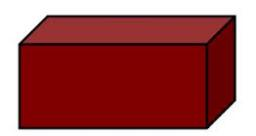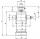# Scale factor

A prism with a volume of 1458 mm3 is scaled down to a volume of 16mm3. What is the scale factor in fraction form?

Correct result:

f =  0.2222

#### Solution:

$f=\sqrt{\frac{16}{1458}}=\frac{2}{9}=0.2222$We would be pleased if you find an error in the word problem, spelling mistakes, or inaccuracies and send it to us. Thank you!Tips to related online calculators
Check out our ratio calculator.
Tip: Our volume units converter will help you with the conversion of volume units.

#### You need to know the following knowledge to solve this word math problem:

We encourage you to watch this tutorial video on this math problem:

## Next similar math problems:

• The volume 2The volume of a cube is 27 cubic meters. Find the height of the cube.
• Cube 6Volume of the cube is 216 cm3, calculate its surface area.
• Prism basesVolume perpendicular quadrilateral prism is 360 cm3. The edges of the base and height of the prism are in the ratio 5:4:2 Determine the area of the base and walls of the prism.
• ScaleCylinder was drawn in scale 2:1. How many times is the volume of the cylinder smaller in reality?
• Area of a cubeCalculate the surface area of a cube if its volume is equal to 729 cubic meters.
• The cubeThe cube has a surface area of 216 dm2. Calculate: a) the content of one wall, b) edge length, c) cube volume.
• TV diagonalDiagonal TV is 0.56 m long, how big the television sreen is if the aspect ratio is 16:9?
• Cube surface areaThe surface of the cube was originally 216 centimeters square. The surface of the cube has shrunk from 216 to 54 centimeters sq. Calculate how much percent the edge of the cube has decreased.
• Cube diagonalsDetermine the volume and surface area of the cube if you know the length of the body diagonal u = 216 cm.
• Center of the cubeThe Center of the cube has a distance 16 cm from each vertex. Calculate the volume V and surface area S of the cube.
• Cube cutIn the ABCDA'B'C'D'cube, it is guided by the edge of the CC' a plane witch dividing the cube into two perpendicular four-sided and triangular prisms, whose volumes are 3:2. Determine in which ratio the edge AB is divided by this plane.
• Cube surface and volumeFind the surface of the cube with a volume of 27 dm3.
• An exampleAn example is playfully for grade 6 from Math and I don't know how to explain it to my daughter when I don't want to use the calculator to calculate the cube root. Thus: A cuboid was made from a block of 16x18x48 mm of modeline. What will be the edge of t
• Prism XThe prism with the edges of the lengths x cm, 2x cm, and 3x cm has volume 20250 cm3. What is the area of the surface of the prism?
• Tetrahedral prismThe height of a regular tetrahedral prism is three times greater than the length of the base edge. Calculate the length of the base edge, if you know that the prism volume is 2187 cm3.
• Cube surface and volumeThe surface of the cube is 500 cm2, how much cm3 will be its volume?
• Body diagonalCalculate the cube volume, whose body diagonal size is 75 dm. Draw a picture and highlight the body diagonal.